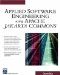# Questions

7.1: Explain how a dynamic scripting language could be implemented using the Beanutils package.

7.2: The batch file extension used to add or manipulate single lined numbers is to be discarded and replaced with a simple single command calculator language. The RPN notation will still exist, but the commands used by the RPN notation will be textual and still operate using the stack principle. The objective is to rewrite the calculator so all commands are dynamic and no command is "hard-coded." Each command consists of key value pairs (e.g., ADD 1234). For the beginning, support the following commands (note that all commands should be stored and read using XML serialization):

ADD: Add the number from the stack.

SUBTRACT: Subtract the number from the stack.

MULTIPLY: Multiply the number from the stack.

DIVIDE: Divide the number from the stack.

STORE: Store the stack value at the identifier specified by the key.

RETRIEVE: Retrieve the value specified by the stack and push it onto the stack.

CLEAR: Clear the stack of all values if the value is 1; otherwise , only clear as many stack positions as indicated by the number.

7.3: Add the instructions to the calculator defined as follows :

BOOKMARKSTART: Defines a starting point for a bookmark.

BOOKMARKEND: Defines an ending point for a bookmark.

SEARCH (e.g., SEARCH COMMANDS\HISTORY\ADD, COMMANDS\ BOOKMARK\SOMETHING): The exact search path used depends on the locally defined object hierarchy. The objective with the SEARCH command is to be able to search a single or multiple commands and then execute those commands in the calculator. Consider this command as a sort of script filter used to execute commands.Applied Software Engineering Using Apache Jakarta Commons (Charles River Media Computer Engineering)
ISBN: 1584502460
EAN: 2147483647
Year: 2002
Pages: 109